# Selina solutions for Concise Maths Class 10 ICSE chapter 15 - Similarity (With Applications to Maps and Models) [Latest edition]

#### Chapters## Solutions for Chapter 15: Similarity (With Applications to Maps and Models)

Below listed, you can find solutions for Chapter 15 of CISCE Selina for Concise Maths Class 10 ICSE.

Exercise 15 (A)Exercise 15 (B)Exercise 15 (C)Exercise 15 (D)Exercise 15 (E)
Exercise 15 (A) [Pages 213 - 215]

### Selina solutions for Concise Maths Class 10 ICSE Chapter 15 Similarity (With Applications to Maps and Models) Exercise 15 (A) [Pages 213 - 215]

Exercise 15 (A) | Q 1.1 | Page 213

In the figure, given below, straight lines AB and CD intersect at P; and AC ‖ BD. Prove that: ΔAPC and ΔBPD are similar.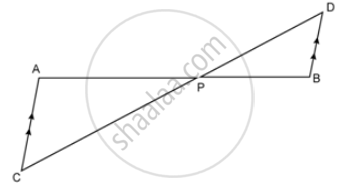Exercise 15 (A) | Q 1.2 | Page 213

In the figure, given below, straight lines AB and CD intersect at P; and AC ‖ BD. Prove that :

If BD = 2.4 cm, AC = 3.6 cm, PD = 4.0 cm and PB = 3.2 cm; find the lengths of PA and PC.Exercise 15 (A) | Q 2.1 | Page 213

In a trapezium ABCD, side AB is parallel to side DC; and the diagonals AC and BD intersect each other at point P. Prove that : Δ APB is similar to Δ CPD.

Exercise 15 (A) | Q 2.2 | Page 213

In a trapezium ABCD, side AB is parallel to side DC; and the diagonals AC and BD intersect each other at point P. Prove that : PA x PD = PB x PC.

Exercise 15 (A) | Q 3.1 | Page 213

P is a point on side BC of a parallelogram ABCD. If DP produced meets AB produced at point L, prove that : DP : PL = DC : BL.

Exercise 15 (A) | Q 3.2 | Page 213

P is a point on side BC of a parallelogram ABCD. If DP produced meets AB produced at point L, prove that : DL : DP = AL : DC.

Exercise 15 (A) | Q 4.1 | Page 213

In quadrilateral ABCD, the diagonals AC and BD intersect each other at point O. If AO = 2CO and BO = 2DO; show that : Δ AOB is similar to Δ COD

Exercise 15 (A) | Q 4.2 | Page 213

In quadrilateral ABCD, the diagonals AC and BD intersect each other at point O. If AO = 2CO and BO = 2DO; show that : OA x OD = OB x OC.

Exercise 15 (A) | Q 5.1 | Page 213

In Δ ABC, angle ABC is equal to twice the angle ACB, and bisector of angle ABC meets the opposite side at point P. Show that : CB : BA = CP : PA

Exercise 15 (A) | Q 5.2 | Page 213

In Δ ABC, angle ABC is equal to twice the angle ACB, and bisector of angle ABC meets the opposite side at point P. Show that : AB x BC = BP x CA

Exercise 15 (A) | Q 6 | Page 213

In ΔABC; BM ⊥ AC and CN ⊥ AB; show that:

(AB)/(AC) = (BM)/(CN) = (AM)/(AN)

Exercise 15 (A) | Q 7.1 | Page 213

In the given figure, DE ‖ BC, AE = 15 cm, EC = 9 cm, NC = 6 cm and BN = 24 cm.

Write all possible pairs of similar triangles.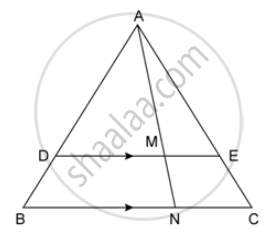Exercise 15 (A) | Q 7.2 | Page 213

In the given figure, DE ‖ BC, AE = 15 cm, EC = 9 cm, NC = 6 cm and BN = 24 cm.

Find lengths of ME and DM.Exercise 15 (A) | Q 8 | Page 213

In the given figure, AD = AE and AD2 = BD x EC.

Prove that: triangles ABD and CAE are similar.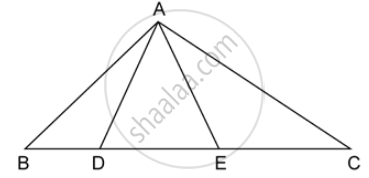Exercise 15 (A) | Q 9 | Page 213

In the given figure, AB ‖ DC, BO = 6 cm and DQ = 8 cm; find: BP x DO.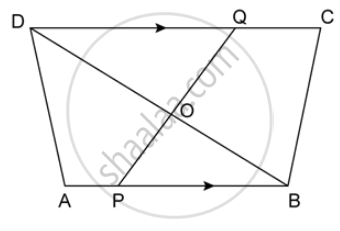Exercise 15 (A) | Q 10 | Page 213

Angle BAC of triangle ABC is obtuse and AB = AC. P is a point in BC such that PC = 12 cm. PQ and PR are perpendiculars to sides AB and AC respectively. If PQ = 15 cm and PR = 9 cm; find the length of PB.

Exercise 15 (A) | Q 11.1 | Page 213

True or False:

Two similar polygons are necessarily congruent.

• True

• False

Exercise 15 (A) | Q 11.2 | Page 213

True or False:

Two congruent polygons are necessarily similar.

• True

• False

Exercise 15 (A) | Q 11.3 | Page 213

True or False:

all equiangular triangles are similar.

• True

• False

Exercise 15 (A) | Q 11.4 | Page 213

True or False:

all isosceles triangles are similar.

• True

• false

Exercise 15 (A) | Q 11.5 | Page 213

True or False:

Two isosceles – right triangles are similar

• True

• false

Exercise 15 (A) | Q 11.6 | Page 213

True or False:

Two isosceles triangles are similar, if an angle of one is congruent to the corresponding angle of the other.

• True

• False

Exercise 15 (A) | Q 11.7 | Page 213

True or False:

The diagonals of a trapezium, divide each other into proportional segments.

• True

• False

Exercise 15 (A) | Q 12 | Page 213

Given:
∠GHE = ∠DFE = 90 ,

DH = 8, DF = 12,
DG = 3x – 1 and DE = 4x + 2.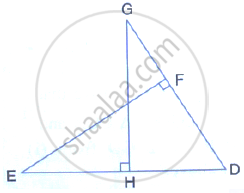Find: the lengths of segments DG and DE

Exercise 15 (A) | Q 13 | Page 213

D is a point on the side BC of triangle ABC such that angle ADC is equal to angle BAC. Prove
that: "CA"^2 = CB × CD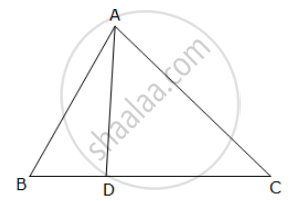Exercise 15 (A) | Q 14 | Page 214

In the given figure,

∆ ABC and ∆ AMP are right-angled at B and M respectively.
Given AC = 10 cm, AP = 15 cm and PM = 12 cm.
(i) Prove ∆ ABC ~ ∆ AMP
(ii) Find AB and BC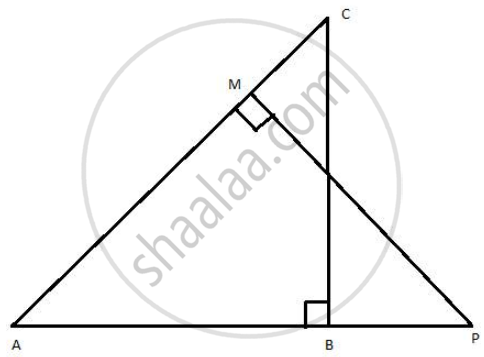Exercise 15 (A) | Q 15 | Page 214

Given: RS and PT are altitudes of ΔPQR. Prove that:
(i) Δ PQT ~ ΔQRS
(ii) PQ × QS = RQ × QT

Exercise 15 (A) | Q 16 | Page 214

Given: ABCD is a rhombus, DRP and CBR are straight lines.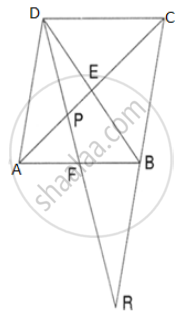Prove that:
DP × CR = DC × PR

Exercise 15 (A) | Q 17 | Page 214

Given: FB = FD, AE ⊥ FD and FC ⊥ AD

Prove: ("FB")/("AD")=("BC")/("ED")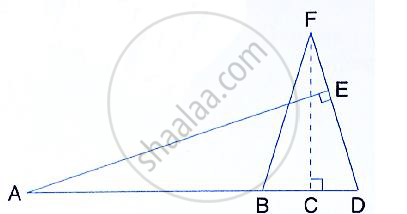Exercise 15 (A) | Q 18 | Page 214

In ∆PQR, ∠Q = 90° and QM is perpendicular to PR. Prove that:
(i) PQ2 = PM × PR

(ii)QR2 = PR × MR

(iii) PQ2 + QR2 = PR2

Exercise 15 (A) | Q 19 | Page 214

In ∆ABC, ∠B = 90° and BD ⊥ AC.
(i) If CD = 10 cm and BD = 8 cm; find AD.
(ii) IF AC = 18 cm and AD = 6cm; find BD.
(iii) If AC = 9 cm and AB = 7cm; find AD.

Exercise 15 (A) | Q 20 | Page 214

In the figure, PQRS is a parallelogram with PQ = 16 cm and QR = 10cm, L is a point on PR
such that RL: LP = 2: 3. QL produced meets RS at M and PS produced at N.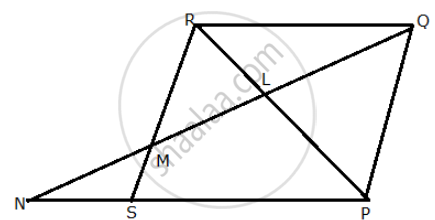Find the lengths of PN and RM.

Exercise 15 (A) | Q 21 | Page 214

In quadrilateral ABCD, diagonals AC and BD intersect at point E such that
AE: EC = BE: ED
Show that ABCD is a  trapezium.

Exercise 15 (A) | Q 22 | Page 214

In triangle ABC, AD is perpendicular to side BC and AD2 = BD × DC.
Show that angle BAC = 90°

Exercise 15 (A) | Q 23 | Page 214

In the given figure, AB ∥ EF ∥ DC ; AB = 67.5 cm, DC = 40.5 cm and AE = 52.5 cm.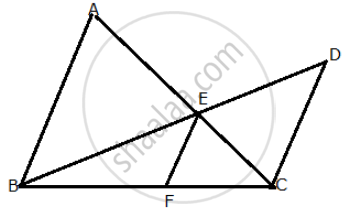(i) Name the three pairs of similar triangles.
(ii) Find the lengths of EC and EF.

Exercise 15 (A) | Q 24 | Page 214

In the given figure, QR is parallel to AB and DR is parallel to AB and DR is parallel to QB.
Prove that: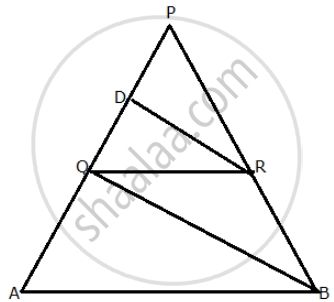PQ^2= PD × PA

Exercise 15 (A) | Q 25 | Page 214

Through the mid-point M of the side CD of a parallelogram ABCD, the line BM is drawn intersecting diagonal AC in L and AD produced in E. Prove that: EL = 2BL.

Exercise 15 (A) | Q 26 | Page 214

In the figure, given below, P is a point on AB such that AP : PB = 4 : 3. PQ is parallel to AC.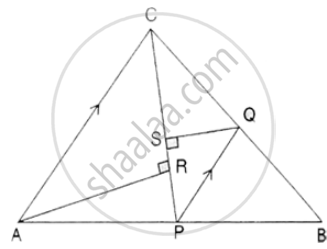(i) Calculate the ratio PQ : AC, giving reason for your answer.
(ii) In triangle ARC, ∠ARC = 90°. Given QS = 6cm, calculate the length of AR.

Exercise 15 (A) | Q 27 | Page 215

In the right-angled triangle QPR, PM is an altitude.
Given that QR = 8cm and MQ = 3.5 cm, calculate the value of PR.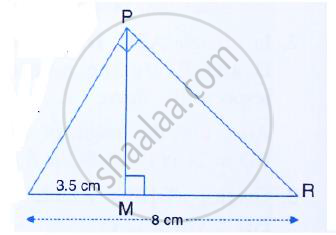Exercise 15 (A) | Q 28 | Page 215

In the figure, given below, the medians BD and CE of a triangle ABC meet at G. Prove that:
(i) Δ EGD ~ ΔCGB and
(ii) BG = 2 GD from (i) above.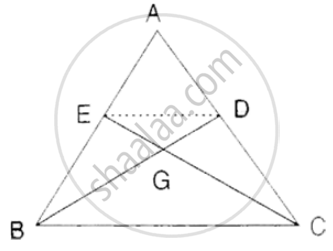Exercise 15 (B) [Page 218]

### Selina solutions for Concise Maths Class 10 ICSE Chapter 15 Similarity (With Applications to Maps and Models) Exercise 15 (B) [Page 218]

Exercise 15 (B) | Q 1.1 | Page 218

In the following figure, point D divides AB in the ratio 3 : 5. Find : (AE)/(EC)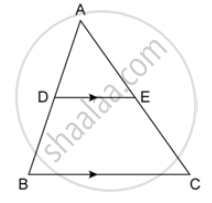Exercise 15 (B) | Q 1.2 | Page 218

In the following figure, point D divides AB in the ratio 3 : 5. Find : (AD)/(AB)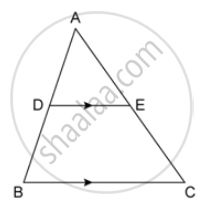Exercise 15 (B) | Q 1.3 | Page 218

In the following figure, point D divides AB in the ratio 3 : 5. Find : (AE)/(AC)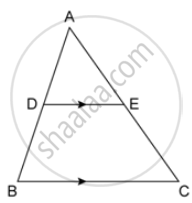Exercise 15 (B) | Q 1.4 | Page 218

In the following figure, point D divides AB in the ratio 3 : 5. Find :

DE = 2.4 cm, find the length of BC.Exercise 15 (B) | Q 1.5 | Page 218

In the following figure, point D divides AB in the ratio 3 : 5. Find :

BC = 4.8 cm, find the length of DE.Exercise 15 (B) | Q 2.1 | Page 218

In the given figure, PQ ‖ AB; CQ = 4.8 cm QB = 3.6 cm and AB = 6.3 cm. Find : (CP)/(PA)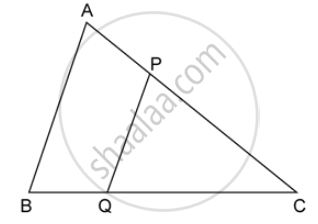Exercise 15 (B) | Q 2.2 | Page 218

In the given figure, PQ ‖ AB; CQ = 4.8 cm QB = 3.6 cm and AB = 6.3 cm. Find :

PQExercise 15 (B) | Q 2.3 | Page 218

In the given figure, PQ ‖ AB; CQ = 4.8 cm QB = 3.6 cm and AB = 6.3 cm. Find :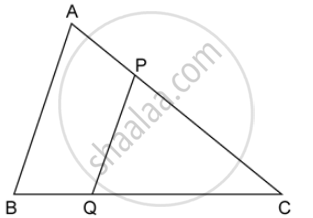If AP = x, then the value of AC in terms of x.

Exercise 15 (B) | Q 3 | Page 218

A line PQ is drawn parallel to the side BC of Δ ABC which cuts side AB at P and side AC at Q. If AB = 9.0 cm, CA = 6.0 cm and AQ = 4.2 cm, find the length of AP.

Exercise 15 (B) | Q 4.1 | Page 218

In Δ ABC, D and E are the points on sides AB and AC respectively.

Find whether DE ‖ BC, if

AB = 9cm, AD = 4cm, AE = 6cm and EC = 7.5cm.

Exercise 15 (B) | Q 4.2 | Page 218

In Δ ABC, D and E are the points on sides AB and AC respectively.

Find whether DE ‖ BC, if AB = 6.3 cm, EC = 11.0 cm, AD =0.8 cm and EA = 1.6 cm.

Exercise 15 (B) | Q 5 | Page 218

In the given figure, Δ ABC ~ Δ ADE. If AE: EC = 4 : 7 and DE = 6.6 cm, find BC. If 'x' be the length of the perpendicular from A to DE, find the length of perpendicular from A to BC in terms of 'x'.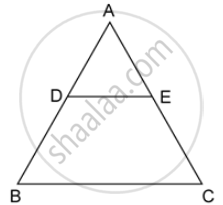Exercise 15 (B) | Q 6 | Page 218

A line segment DE is drawn parallel to base BC of Δ ABC which cuts AB at point D and AC at point E. If AB = 5BD and EC = 3.2 cm, find the length of AE.

Exercise 15 (B) | Q 7 | Page 218

In the figure, given below, AB, CD and EF are parallel lines. Given AB = 7.5 cm, DC = y cm, EF = 4.5 cm, BC = x cm and CE = 3. cm, calculate the values of x and y.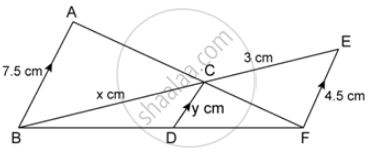Exercise 15 (B) | Q 8 | Page 218

In the figure, given below, PQR is a right-angle triangle right angled at Q. XY is parallel to QR, PQ = 6 cm, PY = 4 cm and PX : XQ = 1 : 2. Calculate the lengths of PR and QR.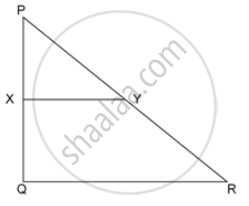Exercise 15 (B) | Q 9 | Page 218

In the following figure, M is mid-point of BC of a parallelogram ABCD. DM intersects the diagonal AC at P and AB produced at E.

Prove that : PE = 2 PD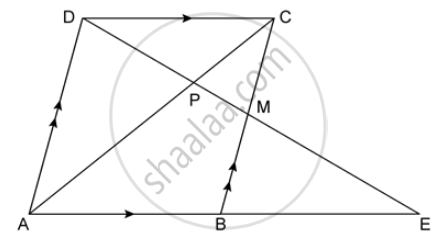Exercise 15 (B) | Q 10 | Page 218

The given figure shows a parallelogram ABCD. E is a point in AD and CE produced meets BA produced at point F. If AE = 4 cm, AF = 8 cm and AB = 12 cm, find the perimeter of the parallelogram ABCD.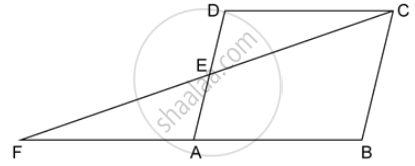Exercise 15 (C) [Page 224]

### Selina solutions for Concise Maths Class 10 ICSE Chapter 15 Similarity (With Applications to Maps and Models) Exercise 15 (C) [Page 224]

Exercise 15 (C) | Q 1.1 | Page 224

The ratio between the corresponding sides of two similar triangles is 2 is to 5. Find the ratio
between the areas of these triangles.

Exercise 15 (C) | Q 1.2 | Page 224

Area of two similar triangles are 98 sq.cm and 128 sq.cm. Find the ratio between the lengths
of their corresponding sides.

Exercise 15 (C) | Q 2 | Page 224

A line PQ is drawn paral el to the base BC of ∆ABC which meets sides AB and AC at points P
and Q respectively. If AP= 1/3PB; find the value of:

1) "Area of ΔABC"/"Area of ΔAPQ"

2)"Area of ΔAPQ"/"Area of trapeziumPBCQ"

Exercise 15 (C) | Q 3 | Page 224

The perimeter of two similar triangles are 30 cm and 24 cm. If one side of the first triangle is 12 cm, determine the corresponding side of the second triangle.

Exercise 15 (C) | Q 4 | Page 224

In the given figure, AX : XB = 3: 5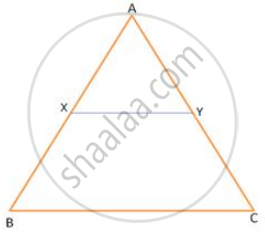(i) the length of BC, if the length of XY is 18 cm.

(ii) the ratio between the areas of trapezium XBCY and triangle ABC.

Exercise 15 (C) | Q 5 | Page 224

ABC is a triangle. PQ is a line segment intersecting AB in P and AC in Q such that PQ ∥ BC and divides triangle ABC into two parts equal in area. Find the value of ratio BP : AB.

Exercise 15 (C) | Q 6 | Page 224

In the given triangle PQR, LM is parallel to QR and PM : MR = 3: 4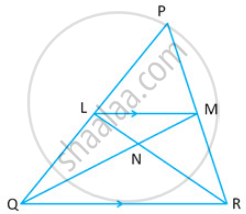Calculate the value of ratio:

1) ("PL")/("PQ") "and then" ("LM")/("QR")

2) "Area of Δ LMN"/"Area of Δ MNR"

3) "Area of Δ LQM"/"Area of Δ LQN"

Exercise 15 (C) | Q 7 | Page 224

The given diagram shows two isosceles triangles which are similar also. In the given diagram,
PQ and BC are not parallel; PC = 4, AQ = 3, QB = 12, BC = 15 and AP = PQ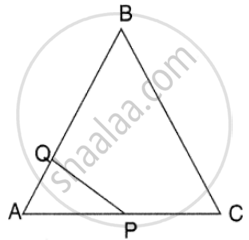Calculate:
(i) the length of AP,
(ii) the ratio of the areas of triangle APQ and triangle ABC.

Exercise 15 (C) | Q 8 | Page 224

In the figure, given below, ABCD is a parallelogram. P is a point on BC such that BP : PC = 1:
2. DP produced meets AB produces at Q. Given the area of triangle CPQ = 20 cm2.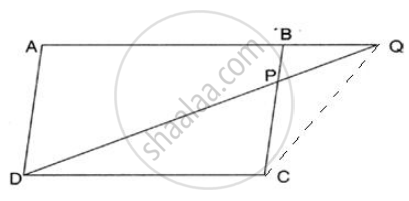Calculate:
(i) area of triangle CDP,
(ii) area of parallelogram ABCD.

Exercise 15 (C) | Q 9 | Page 224

In the given figure, BC is parallel to DE. Area of triangle ABC = 25 cm2, Area of trapezium BCED = 24 cm2 and DE = 14cm.
Calculate the length of BC. Also, find the area of triangle BCD.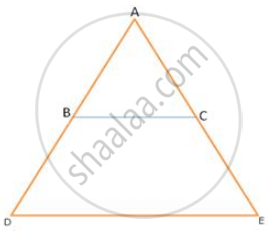Exercise 15 (C) | Q 10 | Page 224

The given figure shows a trapezium in which AB is parallel to DC and diagonals AC and BD
intersect at point P. If AP : CP = 3: 5,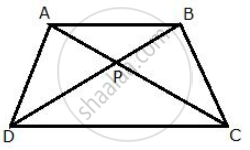Find:
(1) ∆APB : ∆CPB (2 ) ∆DPC : ∆APB

Exercise 15 (C) | Q 11 | Page 224

In the given figure, ABC is a triangle. DE is parallel to BC and ("AD")/("DB")=3/2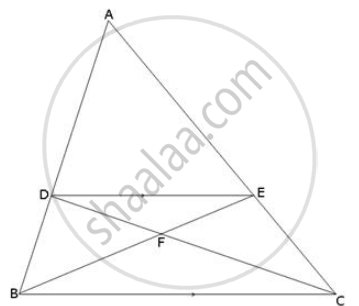(i) Determine the ratios ("AD")/("AB") and ("DE")/("BC")

(ii) Prove that ∆DEF is similar to ∆CBF Hence, find ("EF")/("FB").

(iii) What is the ratio of the areas of ∆DEF and ∆BFC.

Exercise 15 (C) | Q 12 | Page 224

In the given figure, ∠B = ∠E, ∠ACD = ∠BCE, AB = 10.4cm and DE = 7.8 cm. Find the ratio
between areas of the ∆ABC and ∆ DEC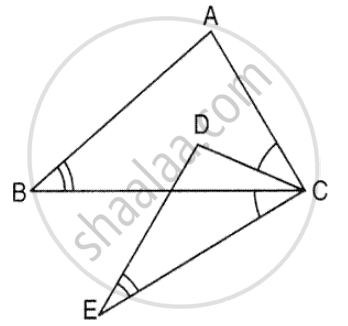Exercise 15 (D) [Page 229]

### Selina solutions for Concise Maths Class 10 ICSE Chapter 15 Similarity (With Applications to Maps and Models) Exercise 15 (D) [Page 229]

Exercise 15 (D) | Q 1.1 | Page 229

A triangle ABC has been enlarged by scale factor m = 2.5 to the triangle A' B' C' Calculate : the length of AB, if A' B' = 6 cm.

Exercise 15 (D) | Q 1.2 | Page 229

A triangle ABC has been enlarged by scale factor m = 2.5 to the triangle A' B' C' Calculate : the length of C' A' if CA = 4 cm.

Exercise 15 (D) | Q 2.1 | Page 229

A triangle LMN has been reduced by scale factor 0.8 to the triangle L' M' N'. Calculate: the length of M' N', if MN = 8 cm.

Exercise 15 (D) | Q 2.2 | Page 229

A triangle LMN has been reduced by scale factor 0.8 to the triangle L' M' N'. Calculate: the length of LM, if L' M' = 5.4 cm.

Exercise 15 (D) | Q 3.1 | Page 229

A triangle ABC is enlarged, about the point O as centre of enlargement, and the scale factor is 3. Find : A' B', if AB = 4 cm.

Exercise 15 (D) | Q 3.2 | Page 229

A triangle ABC is enlarged, about the point 0 as centre of enlargement, and the scale factor is 3. Find : BC, if B' C' = 15 cm.

Exercise 15 (D) | Q 3.3 | Page 229

A triangle ABC is enlarged, about the point 0 as centre of enlargement, and the scale factor is 3. Find : OA, if OA' = 6 cm.

Exercise 15 (D) | Q 3.4 | Page 229

A triangle ABC is enlarged, about the point 0 as centre of enlargement, and the scale factor is 3. Find : OC', if OC = 21 cm

Also, state the value of : (a) (OB')/(OB) (b) (C'A')/(CA)

Exercise 15 (D) | Q 4.1 | Page 229

A model of an aeroplane is made to a scale of 1 : 400. Calculate :

the length, in cm, of the model; if the length of the aeroplane is 40 m

Exercise 15 (D) | Q 4.2 | Page 229

A model of an aeroplane is made to a scale of 1 : 400. Calculate :

the length, in m, of the aeroplane, if length of its model is 16 cm.

Exercise 15 (D) | Q 5 | Page 229

The dimensions of the model of a multistorey building are 1.2 m x 75 cm x 2 m. If the scale factor is 1 : 30; find the actual dimensions of the building.

Exercise 15 (D) | Q 6.1 | Page 229

On a map drawn to a scale of 1 : 2,50,000; a triangular plot of land has the following measurements : AB = 3 cm, BC = 4 cm and  ∠ABC = 90°.

Calculate : the actual lengths of AB and BC in km.

Exercise 15 (D) | Q 6.2 | Page 229

On a map drawn to a scale of 1 : 2,50,000; a triangular plot of land has the following measurements : AB = 3 cm, BC = 4 cm and angle ABC = 90°.

Calculate : the area of the plot in sq. km.

Exercise 15 (D) | Q 7 | Page 229

A model of a ship is made to a scale 1 : 300.

(i) The length of the model of the ship is 2 m. Calculate the length of the ship.

(ii) The area of the deck of the ship is 180,000 m2. Calculate the area of the deck of the model.

(iii). The volume of the model is 6.5 m3. Calculate the volume of the ship.

Exercise 15 (E) [Pages 229 - 232]

### Selina solutions for Concise Maths Class 10 ICSE Chapter 15 Similarity (With Applications to Maps and Models) Exercise 15 (E) [Pages 229 - 232]

Exercise 15 (E) | Q 1.1 | Page 229

In the following figure, XY is parallel to BC, AX = 9 cm, XB = 4.5 cm and BC = 18 cm.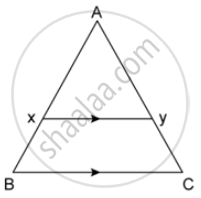(AY)/(YC)

Exercise 15 (E) | Q 1.2 | Page 229

In the following figure, XY is parallel to BC, AX = 9 cm, XB = 4.5 cm and BC = 18 cm.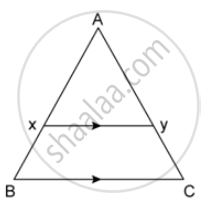(YC)/(AC)

Exercise 15 (E) | Q 1.3 | Page 229

In the following figure, XY is parallel to BC, AX = 9 cm, XB = 4.5 cm and BC = 18 cm.XY

Exercise 15 (E) | Q 2.1 | Page 229

In the following figure, ABCD to a trapezium with AB ‖ DC. If AB = 9 cm, DC = 18 cm, CF= 13.5,cm, AP = 6 cm and BE = 15 cm, Calculate: EC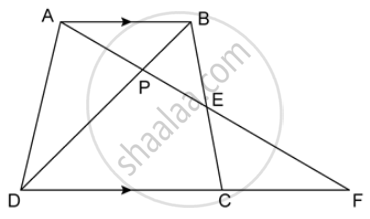Exercise 15 (E) | Q 2.2 | Page 229

In the following figure, ABCD to a trapezium with AB ‖ DC. If AB = 9 cm, DC = 18 cm, CF= 13.5,cm, AP = 6 cm and BE = 15 cm, Calculate: AFExercise 15 (E) | Q 2.3 | Page 229

In the following figure, ABCD to a trapezium with AB ‖ DC. If AB = 9 cm, DC = 18 cm, CF = 13.5,cm, AP = 6 cm and BE = 15 cm, Calculate: PEExercise 15 (E) | Q 3 | Page 229

In the following figure, AB, CD and EF are perpendicular to the straight line BDF.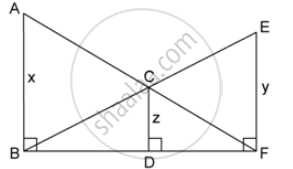If AB = x; CD = z unit and EF = y unit, prove that:

1/"x" + 1/"y" = 1/"z"

Exercise 15 (E) | Q 4 | Page 230

Triangle ABC is similar to triangle PQR. If AD and PM are corresponding medians of the two triangles, prove that: (AB)/(PQ) = (AD)/(PM)

Exercise 15 (E) | Q 5 | Page 230

Triangle ABC is similar to triangle PQR. If AD and PM are altitudes of two triangles, Prove that : (AB)/(PQ) = (AD)/(PM)

Exercise 15 (E) | Q 6 | Page 230

Triangle ABC is similar to triangle PQR. If bisector of angle BAC meets BC at point D and bisector of angle QPR meets QR at point M, prove that  (AB)/(PQ)=(AD)/(PM)

Exercise 15 (E) | Q 7 | Page 230

In the following figure, ∠AXY = ∠AYX. If (BX)/(AX) = (CY)/(AY) show that triangle ABC is isosceles.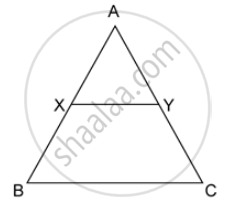Exercise 15 (E) | Q 8 | Page 230

In the given figure, lines l, m and n are such that ls ∥ m ∥ n. Prove that:

(AB)/(BC)=(PQ)/(QR)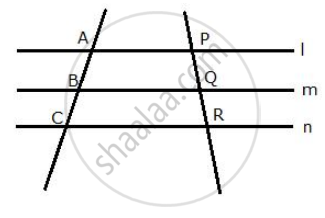Exercise 15 (E) | Q 9 | Page 230

In the following figure, DE || Ac and DC || AP. Prove that: (BE)/(EC) = (BC)/(CP)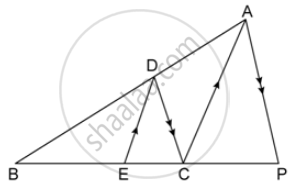Exercise 15 (E) | Q 10.1 | Page 230

In the figure given below, AB ‖ EF ‖ CD. If AB = 22.5 cm, EP = 7.5 cm, PC = 15 cm and DC = 27 cm. Calculate : EF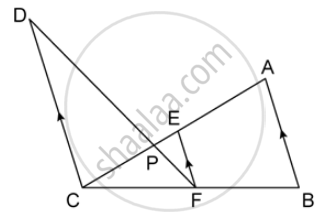Exercise 15 (E) | Q 10.2 | Page 230

In the figure given below, AB ‖ EF ‖ CD. If AB = 22.5 cm, EP = 7.5 cm, PC = 15 cm and DC = 27 cm. Calculate : AC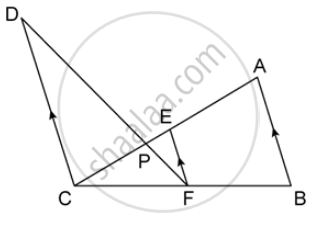Exercise 15 (E) | Q 11 | Page 230

In Δ ABC, ∠ABC = ∠DAC. AB = 8 cm, AC = 4 cm, AD = 5 cm.
1) Prove that ΔACD is similar to ΔBCA.
2) Find BC and CD
3) Find area of ΔACD: area of ΔBCA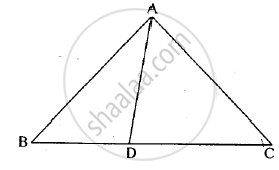Exercise 15 (E) | Q 12 | Page 230

In the given triangle P, Q and R are the mid points of sides AB, BC and AC respectively. Prove that triangle PQR is similar to triangle ABC.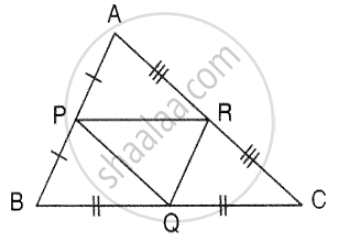Exercise 15 (E) | Q 13 | Page 231

In the following figure, AD and CE are medians of ΔABC. DF is drawn parallel to CE. Prove that :
(i) EF = FB,
(ii) AG : GD = 2 : 1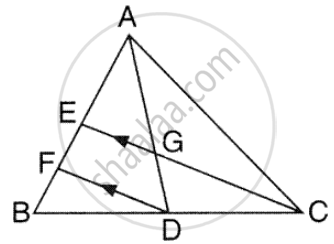Exercise 15 (E) | Q 14 | Page 231

The two similar triangles are equal in area. Prove that the triangles are congruent.

Exercise 15 (E) | Q 15 | Page 231

The ratio between the altitudes of two similar triangles is 3 : 5; write the ratio between their:
(i) medians (ii) perimeters (iii) areas

Exercise 15 (E) | Q 16 | Page 231

The ratio between the areas of two similar triangles is 16 : 25, Find the ratio between their:
(i) perimeters (ii) altitudes (iii) medians

Exercise 15 (E) | Q 17 | Page 231

The following figure shows a triangle PQR in which XY is parallel to QR. If PX : XQ = 1 : 3 and QR = 9 cm. find the length of XY.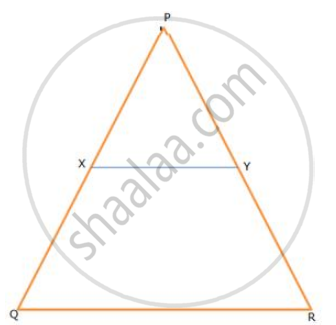Further, if the area of Δ PXY =x cm2 ; find, in terms of x the area of:

(i) triangle PQR (ii) trapezium XQRY

Exercise 15 (E) | Q 18 | Page 231

On a map, drawn to a scale of 1 : 20000, a rectangular plot of land ABCD has AB = 24cm and BC = 32 cm. Calculate:

(i) the diagonal distance of the plot in kilometer

(ii) the area of the plot in sq.km

Exercise 15 (E) | Q 19 | Page 231

The dimensions of the model of a multistoreyed building are 1 m by 60 cm by 1.20 m. if the scale factor is 1 : 50, find the actual dimensions of the building.

Also find:

(i) the floor area of a room of the building, if the floor area of the corresponding room in the model is 50 sq. cm

(ii) the space (volume) inside a room of the model, if the space inside the corresponding room of the building is 90 m^3

Exercise 15 (E) | Q 20 | Page 231

In a triangle PQR, L and M are two points on the base QR, such that ∠LPQ = ∠QRP and ∠RPM

= ∠RQP. Prove that:

(i) ΔPQL and ΔRMP
(ii) QL  xx  RM = P L  ×    PM
(iii)  PQ^2  =   QR  × QL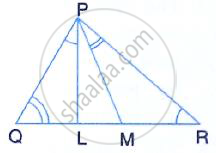Exercise 15 (E) | Q 21 | Page 231

A triangle ABC with AB = 3 cm, BC = 6 cm and AC = 4 cm is enlarged to ΔDEF such that the longest side of ΔDEF = 9 cm. Find the scale factor and hence, the lengths of the other sides of ΔDEF.

Exercise 15 (E) | Q 22 | Page 231

Two isosceles triangles have equal vertical angles. Show that the triangles are similar.If the ratio between the areas of these two triangles is 16 : 25, find the ratio between their corresponding altitudes.

Exercise 15 (E) | Q 23 | Page 231

In ΔABC, AP : PB = 2 : 3. PO is parallel to BC and is extended to Q so that CQ is parallel to BA.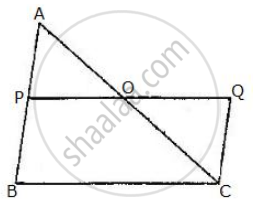Find:

(i) area ΔAPO : area Δ ABC.

(ii) area ΔAPO : area Δ CQO.

Exercise 15 (E) | Q 24 | Page 231

The following figure shows a triangle ABC in which AD and BE are perpendiculars to BC and AC respectively.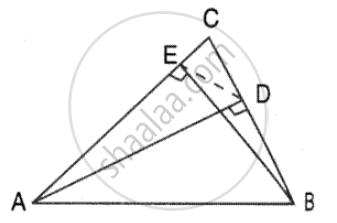Show that;

(ii) CA × CE = CB × CD

(iii) ΔABC ~ ΔDEC

(iv) CD × AB = CA × DE

Exercise 15 (E) | Q 25 | Page 231

In the give figure, ABC is a triangle with ∠EDB = ∠ACB. Prove that ΔABC ~ ΔEBD. If BE =6 cm, EC = 4cm, BD = 5cm and area of ΔBED = 9 cm2. Calculate the:

(i) length of AB]

(ii) area of Δ ABC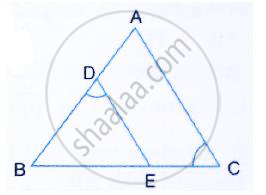Exercise 15 (E) | Q 26 | Page 232

In the given figure, ABC is a right angled triangle with ∠BAC = 90°.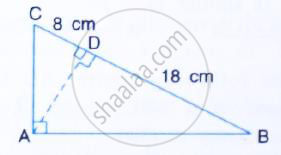(ii) If BD = 18 cm and CD = 8cm, find AD.
(iii) Find the ratio of the area of ΔADB is to area of ΔCDA.

Exercise 15 (E) | Q 27.1 | Page 232

In the given figure, AB and DE are perpendiculars to BC.

Prove that : ΔABC ~  ΔDEC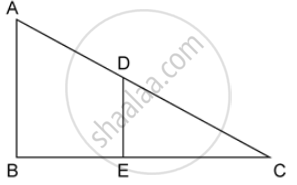Exercise 15 (E) | Q 27.2 | Page 232

In the given figure, AB and DE are perpendiculars to BC.

If AB = 6 cm, DE = 4 cm and AC = 15 cm. Calculate CD.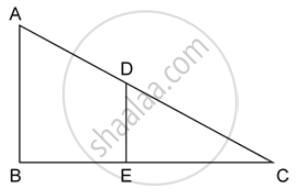Exercise 15 (E) | Q 27.3 | Page 232

In the given figure, AB and DE are perpendiculars to BC.

Find the ratio of the area of a ΔABC : area of ΔDEC.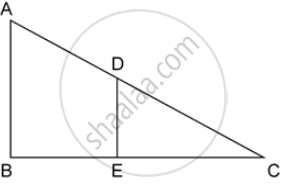Exercise 15 (E) | Q 28.1 | Page 232

ABC is a right angled triangle with ∠ABC = 90°. D is any point on AB and DE is perpendicular to AC. Prove that :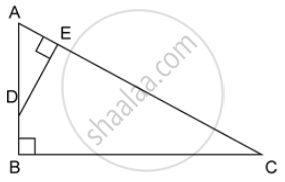Exercise 15 (E) | Q 28.2 | Page 232

ABC is a right angled triangle with ∠ABC = 90°. D is any point on AB and DE is perpendicular to AC. Prove that :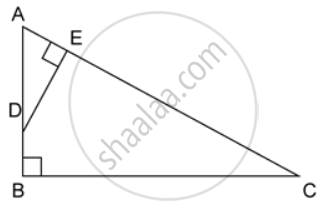If AC = 13 cm, BC = 5 cm and AE = 4 cm. Find DE and AD.

Exercise 15 (E) | Q 28.3 | Page 232

ABC is a right angled triangle with ∠ABC = 90°. D is any point on AB and DE is perpendicular to AC. Prove that :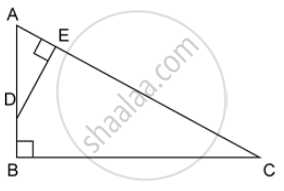Exercise 15 (E) | Q 29 | Page 232

Given: AB || DE and BC || EF. Prove that:

(AD)/(DG)=(CF)/(FG)

 ∆"DFG"  ~ ∆"ACG"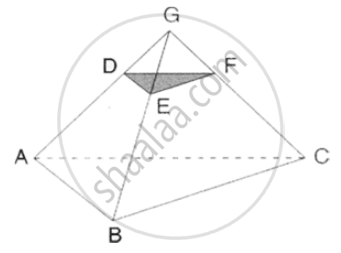Exercise 15 (E) | Q 30 | Page 232

PQR is a triangle. S is a point on the side QR of ΔPQR such that ∠PSR = ∠QPR. Given QP = 8 cm, PR = 6 cm and SR = 3 cm.

1. i. ProveΔPQR∼ Δ
2. Find the lengths of QR and PS.
3. (Area   of DeltaPQR)/(area   of Delta SPR)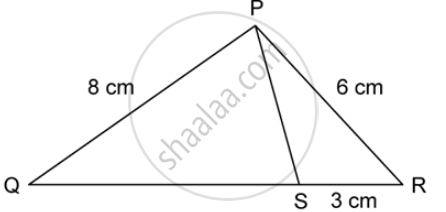## Solutions for Chapter 15: Similarity (With Applications to Maps and Models)

Exercise 15 (A)Exercise 15 (B)Exercise 15 (C)Exercise 15 (D)Exercise 15 (E)## Selina solutions for Concise Maths Class 10 ICSE chapter 15 - Similarity (With Applications to Maps and Models)

Shaalaa.com has the CISCE Mathematics Concise Maths Class 10 ICSE CISCE solutions in a manner that help students grasp basic concepts better and faster. The detailed, step-by-step solutions will help you understand the concepts better and clarify any confusion. Selina solutions for Mathematics Concise Maths Class 10 ICSE CISCE 15 (Similarity (With Applications to Maps and Models)) include all questions with answers and detailed explanations. This will clear students' doubts about questions and improve their application skills while preparing for board exams.

Further, we at Shaalaa.com provide such solutions so students can prepare for written exams. Selina textbook solutions can be a core help for self-study and provide excellent self-help guidance for students.

Concepts covered in Concise Maths Class 10 ICSE chapter 15 Similarity (With Applications to Maps and Models) are Similarity of Triangles, Axioms of Similarity of Triangles, Areas of Similar Triangles Are Proportional to the Squares on Corresponding Sides, Similarity of Triangles.

Using Selina Concise Maths Class 10 ICSE solutions Similarity (With Applications to Maps and Models) exercise by students is an easy way to prepare for the exams, as they involve solutions arranged chapter-wise and also page-wise. The questions involved in Selina Solutions are essential questions that can be asked in the final exam. Maximum CISCE Concise Maths Class 10 ICSE students prefer Selina Textbook Solutions to score more in exams.

Get the free view of Chapter 15, Similarity (With Applications to Maps and Models) Concise Maths Class 10 ICSE additional questions for Mathematics Concise Maths Class 10 ICSE CISCE, and you can use Shaalaa.com to keep it handy for your exam preparation.

Share